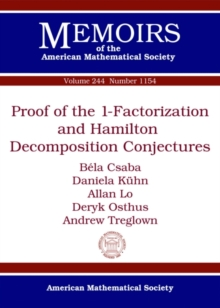Supporting your high street Find out how »
• My Account# Proof of the 1-Factorization and Hamilton Decomposition Conjectures Paperback / softback

## Part of the Memoirs of the American Mathematical Society series

#### Description

In this paper the authors prove the following results (via a unified approach) for all sufficiently large n: (i) [1-factorization conjecture] Suppose that n is even and D 2 n/4 1.

Then every D-regular graph G on n vertices has a decomposition into perfect matchings.

Equivalently, '(G)=D. (ii) [Hamilton decomposition conjecture] Suppose that D n/2 .

Then every D-regular graph G on n vertices has a decomposition into Hamilton cycles and at most one perfect matching. (iii) [Optimal packings of Hamilton cycles] Suppose that G is a graph on n vertices with minimum degree n/2.

Then G contains at least regeven (n, )/2 (n 2)/8 edge-disjoint Hamilton cycles.

Here regeven (n, ) denotes the degree of the largest even-regular spanning subgraph one can guarantee in a graph on n vertices with minimum degree . (i) was first explicitly stated by Chetwynd and Hilton. (ii) and the special case = n/2 of (iii) answer questions of Nash-Williams from 1970.

All of the above bounds are best possible.

#### Information

• Format: Paperback / softback
• Pages: 164 pages
• Publisher: American Mathematical Society
• Publication Date:
• Category: Discrete mathematics
• ISBN: 9781470420253

£89.50

£87.85£84.50

£82.85

£84.50

£82.85

£84.50

£83.89

£84.50

£83.89